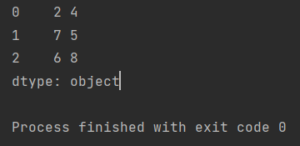Pandas How To Uncategorized How to iterate over rows the Pandas way

# How to iterate over rows the Pandas way

In this lesson I show you how to iterate over rows the Pandas way.

Iterating over rows is possible in many different ways. Python offers many possibilities. This page is dedicated to the Pandas library, so I’ll show you how to iterate over rows using the functions that Pandas offers.

This is my sample dataframe.

```import pandas as pd

my_df = pd.DataFrame({'Column1': ['2','7','6'],
'Column2': ['4','5','8'],
'Column3': ['6','4','3']})

```

## How to iterate over rows

My task will be to iterate over the rows and display the data from the columns with the indexes indicated by me.

I will use the apply method and a lambda expression.

```import pandas as pd

my_df = pd.DataFrame({'Column1': ['2','7','6'],
'Column2': ['4','5','8'],
'Column3': ['6','4','3']})

print(my_df.apply(lambda row: row + " " + row, axis=1))

```With the apply method, the lambda expression was applied to the rows of my dataframe. The for loop iterates my rows and got the expected output.

Here is a link to the apply function documentation.

## Iterating using iterrows method

This method allows you to iterate over the rows of a Pandas DataFrame one at a time and returns an index label for each row and a Series containing the data for that row.

Here is an example of how to use the iterrows() method to iterate over the rows of a Pandas DataFrame:

```import pandas as pd

# create a sample dataframe
df = pd.DataFrame({'name': ['Alice', 'Bob', 'Charlie'], 'age': [25, 30, 35]})

# iterate over the rows of the dataframe
for index, row in df.iterrows():
print(index, row['name'], row['age'])```

In this example, we first create a sample DataFrame with two columns ‘name’ and ‘age’. Then, we use the iterrows() method to iterate over the rows of the DataFrame. The iterrows() method returns an iterator that yields pairs of index and row data as Series. In the loop, we print the index and the values of the ‘name’ and ‘age’ columns for each row.

It is worth noting that iterating over rows using iterrows() can be slower than other vectorized operations that Pandas offers. If possible, it is recommended to use vectorized operations or other methods like apply() or applymap() to perform operations on a DataFrame.

Tags: ,

## How to print column namesHow to print column names

In today’s post you will learn how to print column names from your dataframe in Pandas.

## How to replace values in a columnHow to replace values in a column

Working with data is not easy. Not only you have to prepare, clean and interpret them, you also often have to correct the data. This is a tutorial on how

## How to solve RuntimeError in PandasHow to solve RuntimeError in Pandas

A RuntimeError in Pandas typically occurs when there is an error in your code related to the execution of the program.